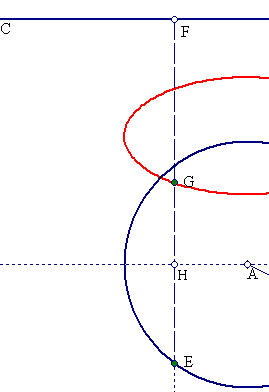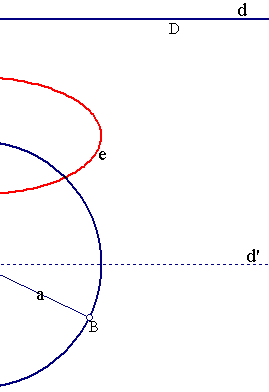##Ellipse construction

Consider a circle c(A,a) and a point E moving on it. Project E to a point F on a line d = (CD). Define point G on e = (EF) s.t. the length-ratio GF/EF is equal to b/a, b a constant 0 <b <a, as E moves on c. Then G describes an ellipse (e) with axes a and b.The statement is a consequence of the discussion in Auxiliary.html . There the property is proved for the special location of the line CD in which it coincides with a diameter of the circle. For the general case draw a line d' parallel to d, passing through the center A. Then the requirement GF/EF = b/a = > GF = EF*(b/a) = (EH+HF)*(b/a) = EH*(b/a) + HF*(b/a) = EH*(b/a) + m, where m = HF*(b/a) is independent of the location of E. On the other side EH*(b/a) defines on HE a point of the ellipse (e') with axes a, b, having the great axis a along d'. The ellipse (e) is a translation of (e') through the constant vector m.

A related construction of the ellipse is discussed in the file Ellipse_Construction.html .

Switch to the [Select-on-contour] tool (CTRL+2) and pick-move point G to see how the shape of the ellipse changes, in dependence of the position of G on EF.
Look at Foci_on_ellipse.html for the locus of the focal points of the various ellipses, as G moves on the line EF.

 Produced with EucliDraw© http://users.math.uoc.gr/~pamfilos/